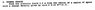# Solve Sphere Charge: Find Electric Field 2.5m from Center

• stumpoman

## Homework Statement

I have a question about notation. My professor posted an older practice test with some different notation techniques than I am used to.

"Sphere Charge
Find the electric field 2.5 m from the center of a region of space with a charge density given by ro=5.5 E-15 R**(2.3)"

I understand most of it but am unsure what the R**(2.3) means.

E = kQ/r^2
ρ = 5.5 E-15

## The Attempt at a Solution

I assume the R**(2.3) has something to do with the volume of the sphere but other than that I have nothing.

Attached is a screenshot of the question.

#### Attachments

•Sphere Charge.jpg
8.3 KB · Views: 287

## Homework Statement

I have a question about notation. My professor posted an older practice test with some different notation techniques than I am used to.

"Sphere Charge
Find the electric field 2.5 m from the center of a region of space with a charge density given by ro=5.5 E-15 R**(2.3)"

I understand most of it but am unsure what the R**(2.3) means.

E = kQ/r^2
ρ = 5.5 E-15

## The Attempt at a Solution

I assume the R**(2.3) has something to do with the volume of the sphere but other than that I have nothing.

Attached is a screenshot of the question.

'**' is Fortran talk for power. It's just ##R^{2.3}##. It's giving the charge density as a function of distance from the center of the sphere. You need to integrate that over the sphere to get total charge.

•1 person